sbi clerk :: numerical ability :: test 8

Home sbi clerk / numerical ability Questions and Answers

1.  Directions(Q.1 to Q.6 ):
Given below, the table which shows the total students in 4 different class of a school and percentage of students participating in Dance and play from these 4 classes.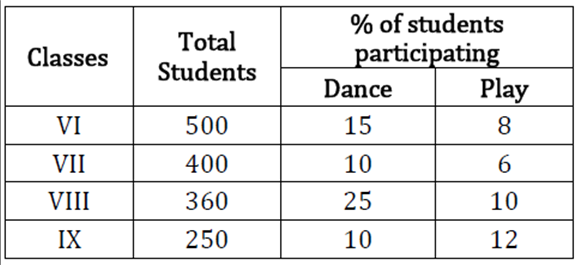What is the ratio between students participating in Dance from Class VII and IX together to the students participating in Play from class VI and VIII together

a

43 : 53

b

65 : 76

c

44 : 57

d

63 : 71

e

62 : 77View AnswerDiscuss
 Answer : Option B Explanation : Required ratio = $= \frac{{\frac{{10}}{{100}} \times 400 + \frac{{10}}{{100}} \times 250}}{{\frac{8}{{100}} \times 500 + \frac{{10}}{{10}} \times 360}} = 65:76$

2.  What is the average of students who participating in Play from all class.

a

$32\frac{1}{2}$

b

$34\frac{1}{2}$

c

$27\frac{1}{2}$

d

$35\frac{1}{2}$

e

$30\frac{1}{2}$View AnswerDiscuss
 Answer : Option A Explanation : Required average = $\frac{{\frac{8}{{100}} \times 500 + \frac{6}{{100}} \times 400 + \frac{{10}}{{100}} \times 360 + \frac{{12}}{{100}} \times 250}}{4}$ $= \frac{{130}}{4} = \frac{{65}}{2} = 32\frac{1}{2}$

3.  Students who are participating in dance from class VII are what percent more or less than students who are participating is play from class IX.

a

$12\frac{2}{7}{\rm{\% }}$

b

$14\frac{2}{7}{\rm{\% }}$

c

$33\frac{1}{3}{\rm{\% }}$

d

$16\frac{2}{3}{\rm{\% }}$

e

$66\frac{2}{3}{\rm{\% }}$View AnswerDiscuss
 Answer : Option C Explanation : Students participating in dance from Class VII $= \frac{{10}}{{100}} \times 400 = 40$ Students participating in play from class IX $= \frac{{12}}{{100}} \times 250 = 30$ Required percentage $= \frac{{10}}{{30}} \times 100 = \frac{{100}}{3}{\rm{\% }} = 33\frac{1}{3}{\rm{\% }}$

4.  What is the sum of students who does not participate in dance and play from class VI and IX together.

a

720

b

480

c

620

d

580

e

560View AnswerDiscuss
 Answer : Option D Explanation : Students who don’t participate in dance and play from class VI =500−(15%+8%)???????? 500 =500− $\frac{{23}}{{100}} \times 500$ =500−115 =385 Students who do not participate in dance and play from class IX =250−(10%+12%)×250 =250−55 = 195 Required sum = 195 + 385 = 580

5.  If 20% of students who participate in dance from class VI also participate in play then find the ratio of students from class VI who participated only in Dance to students participated only in play.

a

12 : 5

b

16 : 25

c

19 : 20

d

20 : 19

e

15 : 11View AnswerDiscuss
 Answer : Option A Explanation : Students who participate only in dance from class VI $= \frac{{15}}{{100}} \times 500 - \frac{{20}}{{100}} \times \frac{{15}}{{100}} \times 500$ $= 75 - \frac{1}{5} \times 75 = 60$ Students who participate only in play from class VI $= \frac{8}{{100}} \times 500 - 15 = 40 - 15 = 25$ Required ratio = 60 : 25 = 12 : 5

6.  Students participating in Dance from class VII is what percent of students participating in play from class IX.

a

$33\frac{1}{3}{\rm{\% }}$

b

$120\frac{2}{7}{\rm{\% }}$

c

$114\frac{2}{7}{\rm{\% }}$

d

$133\frac{1}{3}{\rm{\% }}$

e

$116\frac{2}{3}{\rm{\% }}$View AnswerDiscuss
 Answer : Option D Explanation : Required ratio $= \frac{{\frac{{10}}{{100}} \times 400}}{{\frac{{12}}{{100}} \times 250}} \times 100$ $= \frac{{10 \times 400}}{{12 \times 250}} \times 100 = 133\frac{1}{3}{\rm{\% }}$

7.  Directions(Q.7 to Q.11 ):
What should come in place of question mark (?) in the following number series?

3, 5, 15, 45, 113, ?

a

190

b

234

c

293

d

243

e

208View AnswerDiscuss
 Answer : Option D Explanation :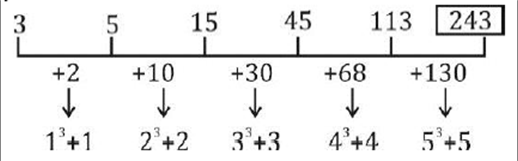8.  17, 98, 26, 89, 35, ?

a

78

b

79

c

80

d

81

e

82View AnswerDiscuss
 Answer : Option C Explanation :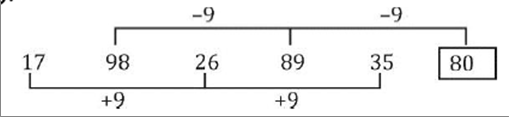9.  3240, 540, 108, 27, ?, 4.5

a

12

b

7

c

9

d

8

e

6View AnswerDiscuss
 Answer : Option C Explanation :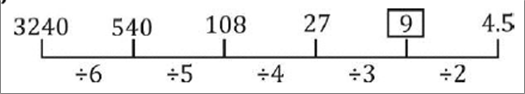10.  7, 4.5, 5.5, 12, 49, ?

a

393

b

378

c

197

d

148

e

246View AnswerDiscuss
 Answer : Option A Explanation :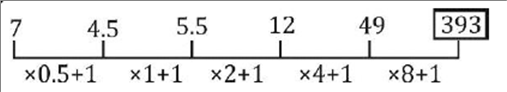11.  2, 17, 89, 359, 1079, ?

a

2134

b

1081

c

2195

d

2159

e

1945View AnswerDiscuss
 Answer : Option D Explanation :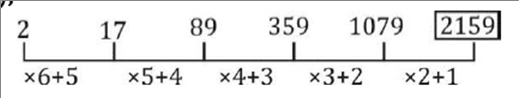In some shifts 5 in quality asked in place of series.

12.  Directions(Q.17 to Q.23 ):
Find out of the approximate value of ? in the following questions

$\left( {\sqrt {80.997} - \sqrt {25.001} } \right) \times (\sqrt {120.90} + \sqrt {16.02)} = ?$

a

50

b

60

c

75

d

70

e

55View AnswerDiscuss
 Answer : Option B Explanation : ≈ (9 – 5) × (11 + 4) = ? ≈ ? = 60

13.  55.01 – 345.02 ÷ 22.99 = 2×?

a

20

b

25

c

22

d

15

e

18View AnswerDiscuss
 Answer : Option A Explanation : $\approx 55 - \frac{{345}}{{23}} = 2 \times ? \approx ? = 20$

14.  $\sqrt {3099.985 \div 62.001 + 14.001} = ?$

a

4

b

8

c

6

d

9

e

5View AnswerDiscuss
 Answer : Option B Explanation : $\begin{array}{*{20}{c}} { \approx \sqrt {\frac{{3100}}{{62}} + 14} }\\ { \approx \sqrt {50 + 14} \approx 8} \end{array}$

15.  (111.99×5)÷14.02=11.002+ ?

a

34

b

19

c

39

d

29

e

38View AnswerDiscuss
 Answer : Option D Explanation : ≈(112×5)÷14=11+ ? ≈40−11= ? ≈ ?=29Numerical AbilityEnglish LanguageReasoning
Copyright 2021 - Govt Exam Guru Made withfor Govt Jobs Aspirants. | Privacy Policy | Terms and Conditions | Contact us | Sitemap |XML